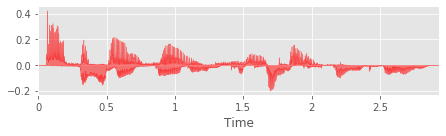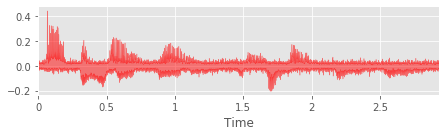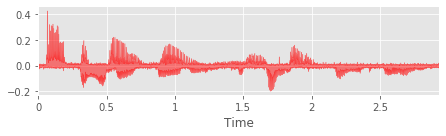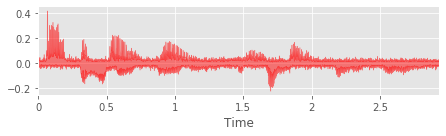# Custom Transformations¶

In this notebook we will go over the basics of creating a custom transformation in Sigment, using the Transform base class.

To best understand this notebook, it is recommended that you read the Using Transformations notebook first.

Contents:

In :
import matplotlib.pyplot as plt
from sigment import Transform
from utils import plot
plt.style.use('ggplot')


## General structure of a custom transformation class¶

A general custom transformation will be a subclass of the Transform base class, with the following structure:

class CustomTransform(Transform):
def __init__(self, *params, p=1., random_state=None):
super().__init__(p, random_state)
# 1. Validate and set your parameters as instance variables here

def _transform(self, X, sr):
X = self._val.signal(X)

# 2. Validate the sample rate if it is required for your transformation
sr = self._val.restricted_integer(sr, 'sr (sample rate)', lambda x: x > 0, 'positive')

# 3. Sample values for your parameters here
# 4. Apply the transformation to the signal here
return X


The validations used in step 1 depend on the nature of the parameters used for the transformation, but these will normally be one of the float_value, integer_value, one_of, or boolean validations from the internal validations class.

If the transformation you are applying does not rely on the sample rate, then step 2 is not necessary.

In step 3, sampling from the value range of each parameter is performed. For a parameter param, assuming the self.param value range was set in step 1, the following should be performed in this step (for each parameter):

# If the parameter is discrete (an integer)
param = self.random_state.randint(self.param, self.param + 1)
# If the parameter is continuous (a real number)
param = self.random_state.uniform(*self.param)


The transformation applied in step 4 should then use the new sampled parameter values.

## Implementing a custom Laplacian white noise transformation¶

To demonstrate how to create a custom transformation class, we will now implement an additive Laplacian white noise transformation.

### The Laplace distribution¶

The Laplace distribution is a continuous probability distribution that can be used to generate signal noise. The probability density function is given by:

$$p(x\ |\ \mu, b) = \frac{1}{2b} \exp\left(-\frac{|x - \mu|}{b}\right)$$

where $\mu\in\mathbb{R}$ and $b\in\mathbb{R}^+$ are the location and scale parameters, respectively.

As with additive Gaussian white noise we set $\mu=0$ and vary $b$.

With a signal $\mathbf{x}=x_1,x_2,\ldots,x_T$ and noise $\mathbf{n}=n_1,n_2,\ldots,n_T$ where $n_i\sim\text{Laplace}(0, b)$, the signal with added noise is given by $\mathbf{x}' = \mathbf{x} + \mathbf{n}$.

### Defining the transformation class¶

We will now define a LaplacianWhiteNoise transformation class to apply additive Laplacian white noise to a signal.

In :
class LaplacianWhiteNoise(Transform):
"""Applies additive Laplacian white noise to the signal."""

def __init__(self, scale, p=1., random_state=None):
super().__init__(p, random_state)

# 1. Validate and set your parameters as instance variables here
# As scale is real-valued, we use the float_value validator
self.scale = self._val.float_value(
scale, 'scale (scale parameter)',
lambda a, b: 0. < a <= b, 'positive')

def _transform(self, X, sr):
X = self._val.signal(X)

# 2. Validate the sample rate if it is required for your transformation
# Not necessary for this transformation

# 3. Sample values for your parameters here
# As scale is real-valued, we sample from a uniform distribution
scale = self.random_state.uniform(*self.scale)

# 4. Apply the transformation to the signal here
noise = self.random_state.laplace(loc=0, scale=scale, size=X.shape)
return X + noise


To observe the effects of the transformation we just created, we will load an audio signal from a sample WAV file and apply the transformation.

In :
X, sr = load('assets/audio.wav', mono=False)
plot(X, sr=sr)### Testing the new transformation class¶

In :
transform = LaplacianWhiteNoise(scale=(0.001, 0.01), random_state=0)
for X_aug in transform.generate(X, n=3):
plot(X_aug, sr=sr)